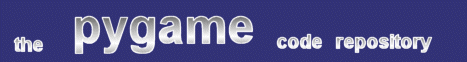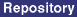### Title: Zoom-able Surface Class

Author: David Rooney (idfx at hotmail.com)
Submission date: Feb 8, 2002

Description: A surface class that draws itself to user-supplied dimensions.

pygame version required: Pygame 1.4 Feb 8 CVS, or newer
SDL version required: Any
Python version required: 2.2 or newer

Comments: Traditionally, it has been impossible to subclass fundamental classes like pygame's Surface class. This has meant that if you wanted to implement a varient of the Surface class, you had to wrap it; this took more effort and introduced overhead for each method call. With Python 2.2, however, you can inherit from base classes, and with February 8th's CVS commit, you can subclass the Surface and Rect classes.

You can use the ZoomSurface just like a regular surface, following the instructions mentioned in the comments below. ZoomSurfaces have one additional power - the 'output' drawing can larger or smaller than the actual surface dimensions. For example, your surface might be 320 x 240, and you could have it drawn at 640 x 480, so small details can be more easily seen. Normally, you'd have to do a pygame.transform call yourself to accomplish this, but ZoomSurfaces do it automatically.

```
#! /usr/bin/env python
#
#		ZoomScreen.py
#
#

import pygame
from pygame.constants import HWSURFACE, RESIZABLE, ASYNCBLIT, OPENGL, HWPALETTE, DOUBLEBUF, FULLSCREEN, RLEACCEL
from pygame import display
from pygame.transform import scale, rotozoom
from math import tan, pi

class ZoomScreen:

"""
"""

def __init__(self, size, source = None, opts = DOUBLEBUF):

"""		ZoomScreen( size [,source [,options]] )   -->   None

This is intended to be the main screen, the one usually returned
by display.set_mode()
Therefore, it takes mostly the same arguments as set_mode()does, with
the optional addition of a specified source surface.

The source surface defaults to None, which is really useless unless you
set_source() soon after...

This was written mainly with the intent of making small-scale graphics
work visible while debugging, although I think it could also be used
to show a miniature of a large surface, instead.  (eg: an inset map of
the whole world while one sector of it is on screen)

Possibly useless technical note:  if given a tuple, this function will
use the transform.scale() function, or if given a scaling factor, will
use tranform.rotozoom() (with rotation==0)
"""
pygame.init()
try:
if len(size) == 2:
self.size = size
elif len(size) == 4:
self.size == size[-2:]
else:
self.size = size[:2]
except TypeError:
self.size = float(size)
self.img = display.set_mode( self.size, opts )
if source:
self.source = source
else:
self.source = Surface( (0,0) )

def set_source(self, src):
if type(src) == pygame.SurfaceType:
self.source = src
self.draw()
return

def draw(self):

"""		ZoomScreen.draw()   -->  None
"""
try:
len(self.size)
self.img.blit( scale(self.source, self.size),(0,0) )
except TypeError:
self.img.blit( rotozoom(self.source, 0, self.size),(0,0) )
return

class ZSurface( pygame.Surface ):

"""		Mostly like any other Surface, with a few extra methods for zoom-related
actions.  Scales the ZSurface to any arbitrary size requested, when drawing.

The ZSurface itself remains a constant size, acting just like a normal Surface;
it only scales its draw() output.

New member variables are:
zoomsize 				# most recent requested size
_aspect_ratio			# (read-only) current aspect ratio

Any Surface inquiries having to do with size will answer with actual sizes,
not zoomed ones.
"""

def __init__(self, *argv):
Surface.__init__(self, argv)
self.zoomsize = None
if self.get_height() != 0:
self._aspect_ratio = float(self.get_width()) / float(self.get_height())
else:
self._aspect_ratio = None

def get_aspect_ratio(self):

"""		ZSurface.get_aspect_ratio()  -->  float or None

This method returns this ZSurface's aspect ratio, which is its width divided
by its height ( w/h ).  If height is zero, (ie, divide-by-zero trouble) the
aspect ratio is redefined as None.
"""
return self._aspect_ratio

def check_ratio(self, src, acceptable = 0.1):

"""		ZSurface.check_ratio( src [, acceptable_distortion] )  -->  int

Designed as a small convenience, this method will compare the aspect
ratios of this ZSurface and the given src Surface.  If the difference
between them is a float within an acceptable range, 0.1 (10%) by
default, this returns a 1, giving back a 0 if the distortion is too
great.

If either Surface has an aspect ratio of None, it's certainly a bad
match, so it will simply return 0.

This function is best called _before_ blitting a Surface to this one.
Otherwise, you could mistakenly blit a 10x75 Surface into, say, a
320x480 ZSurface, with appropriately disasterously ugly stretchy
results.
However, squeezing a 180x200 Surface into a 170x190 ZSurface shouldn't
work out too badly (it's less than 1% distortion).

Note that this function won't prevent you from doing such a (possibly)
foolish thing, if you want to.  Its sole purpose is to check beforehand.
"""
if self._aspect_ratio == None:
return 0
else:
if is_instance( src, ZSurface ):
othrAR = ZSurface.get_aspect_ratio()
if othrAR == None:
return 0
else:
x, y = src.get_size()
if y== 0 or x==0:
return 0
else:
othrAR = float(x)/float(y)
ratio_diff = self._aspect_ratio - othrAR
if abs( ratio_diff ) <= acceptable:
return 1
else:
return 0

def draw(self, (wide,high)=(0,0) ):

"""		ZSurface.draw( [ (width,height)=(0,0) ] )    -->  Surface

This function is for output only.  Big surprize.

Called without arguments, it will return a copy of this ZSurface
in its last known dimensions.  That is, if you haven't ever resized it,
it will return in original size, but if you asked it to draw itself at,
say, 320x200, each subsequent call will return a Surface of 320x200,
until you specify otherwise.

This method will optionally take 1 argument, a length==2 sequence to
designate the desired return size (which will then be its default size).

In the future, I think I'll also make it support RectType arguments, but
it doesn't yet.  Sorry.
"""
if (wide == 0) and (high == 0):
if self.zoomsize:
zsize = self.zoomsize
else:
zsize = self.get_size()
elif (wide == 0) or (high == 0):
if self.zoomsize:
if wide == 0:
wide == self.zoomsize
else:
high == self.zoomsize
self.zoomsize = [wide,high]
else:
if wide == 0:
wide == self.get_width()
else:
high == self.get_height()
self.zoomsize = [wide,high]
zsize = self.zoomsize
else:
self.zoomsize = [wide,high]
zsize = None
if zsize:
return scale( self, zsize )
else:
return self

```

 Feedback